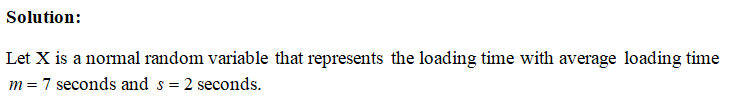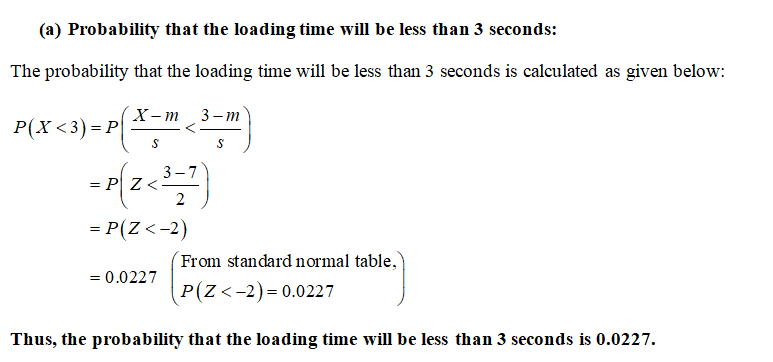# It is known that the “Stats is Awesome” webpage takes on average 7 seconds to load (m = 7) with a population standard deviation of 2 seconds (s = 2). (a) What is the probability that the loading time will be less than 3 seconds?            (b) What is the probability that the loading time will be between 6 and 9 seconds?

Question
4 views

It is known that the “Stats is Awesome” webpage takes on average 7 seconds to load (m = 7) with a population standard deviation of 2 seconds (s = 2).

(a) What is the probability that the loading time will be less than 3 seconds?

(b) What is the probability that the loading time will be between 6 and 9 seconds?

check_circle

Step 1Step 2...

### Want to see the full answer?

See Solution

#### Want to see this answer and more?

Solutions are written by subject experts who are available 24/7. Questions are typically answered within 1 hour.*

See Solution
*Response times may vary by subject and question.
Tagged in